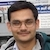# NAEST 2014 Screening Test: Wheatstone BridgeBy

In screening round of National Anveshika Experimental Skills Test ( NAEST 2014 ), a question was asked on the five electric bulb. In the second half of video, the bulb at the middle stops glowing when two bulbs are interchanged.

Question: Why bulb at centre stopped glowing when two bulbs are interchanged?

Answer: This video demonstrates balanced Wheatstone bridge using 5 bulbs - two 100 W bulbs, two 60 W bulbs and a 10 W bulb (at centre). Let two types of peripheral bulbs have resistances $R_1$ and $R_2$ and central bulb is of resistance $R$.

In first case, the bridge was not balanced giving non-zero current through $R$. The 10 W bulb at the centre glows in this case.

In second case, the bridge is balanced giving zero current through $R$. The bulb stops glowing in this case. These two cases are shown in figure.